Скачать презентацию FMPro Finds and Calculations Giving FM a brain

0e25bfbc2b89c346586145fb829d0a16.ppt

• Количество слайдов: 34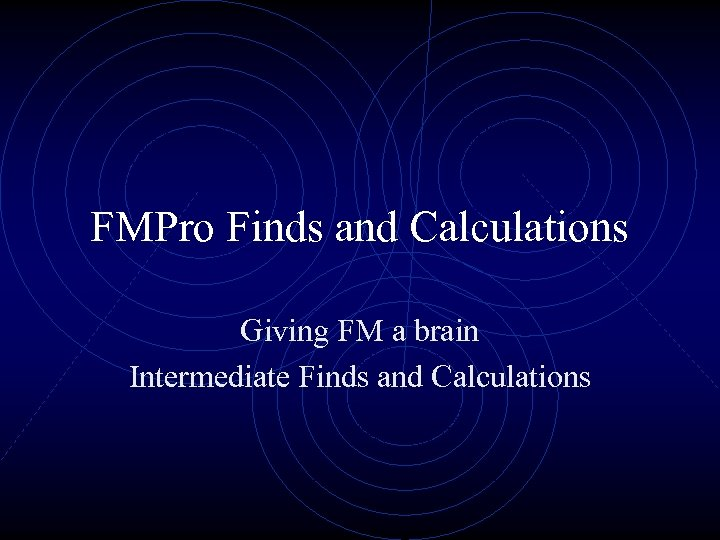FMPro Finds and Calculations Giving FM a brain Intermediate Finds and Calculations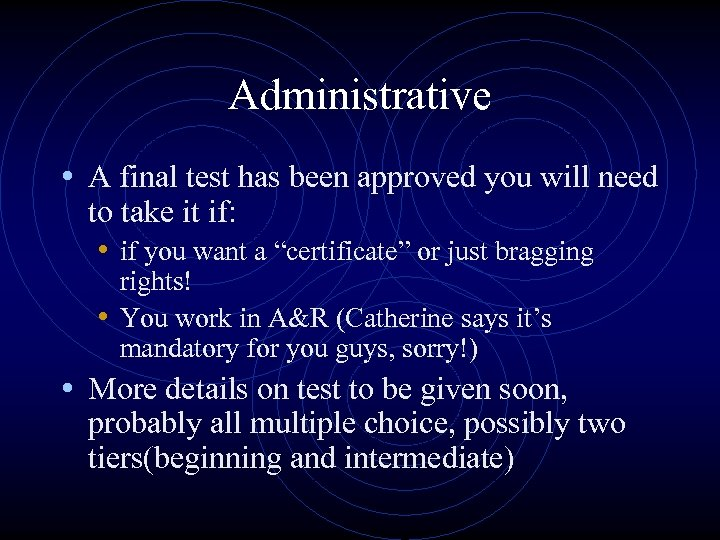Administrative • A final test has been approved you will need to take it if: • if you want a “certificate” or just bragging rights! • You work in A&R (Catherine says it’s mandatory for you guys, sorry!) • More details on test to be given soon, probably all multiple choice, possibly two tiers(beginning and intermediate)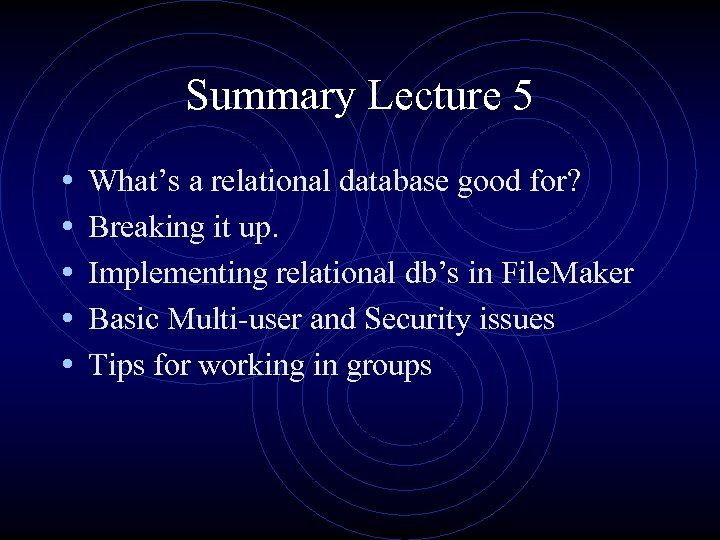Summary Lecture 5 • • • What’s a relational database good for? Breaking it up. Implementing relational db’s in File. Maker Basic Multi-user and Security issues Tips for working in groups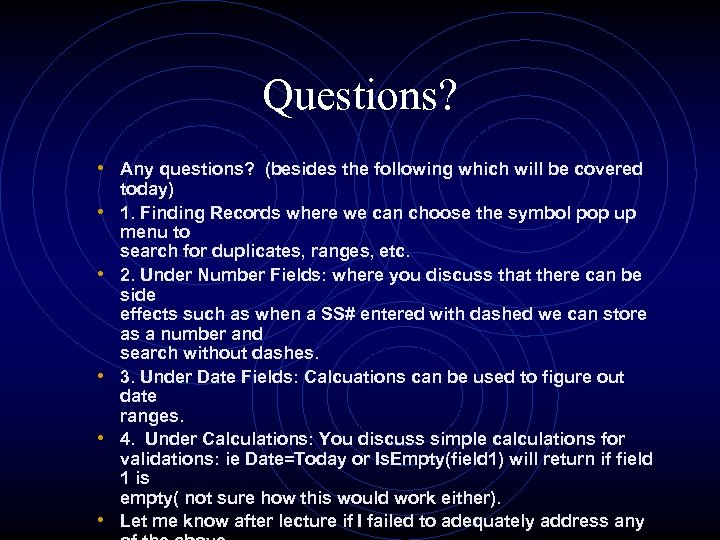Questions? • Any questions? (besides the following which will be covered • • • today) 1. Finding Records where we can choose the symbol pop up menu to search for duplicates, ranges, etc. 2. Under Number Fields: where you discuss that there can be side effects such as when a SS# entered with dashed we can store as a number and search without dashes. 3. Under Date Fields: Calcuations can be used to figure out date ranges. 4. Under Calculations: You discuss simple calculations for validations: ie Date=Today or Is. Empty(field 1) will return if field 1 is empty( not sure how this would work either). Let me know after lecture if I failed to adequately address any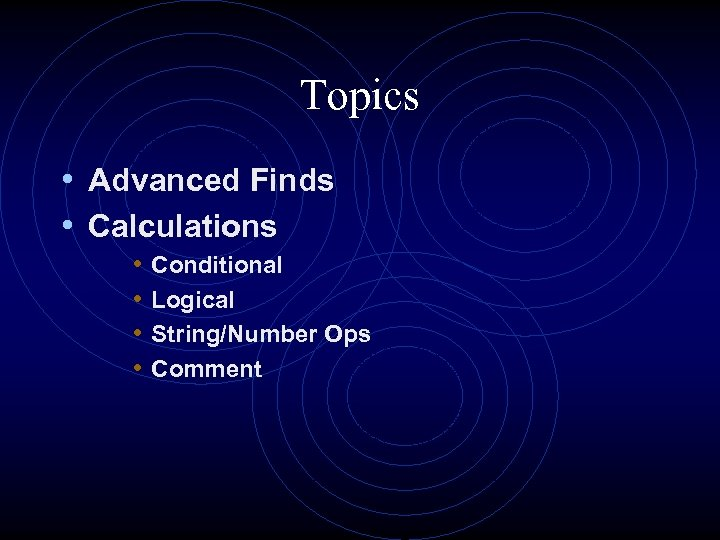Topics • Advanced Finds • Calculations • • Conditional Logical String/Number Ops Comment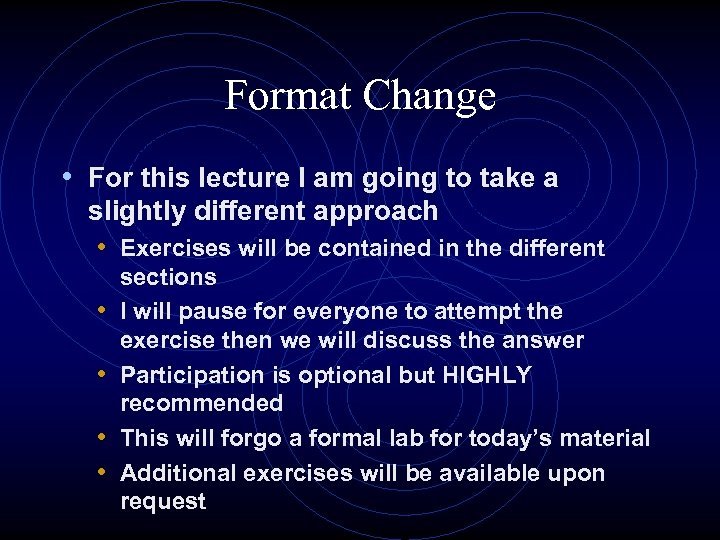Format Change • For this lecture I am going to take a slightly different approach • Exercises will be contained in the different • • sections I will pause for everyone to attempt the exercise then we will discuss the answer Participation is optional but HIGHLY recommended This will forgo a formal lab for today’s material Additional exercises will be available upon request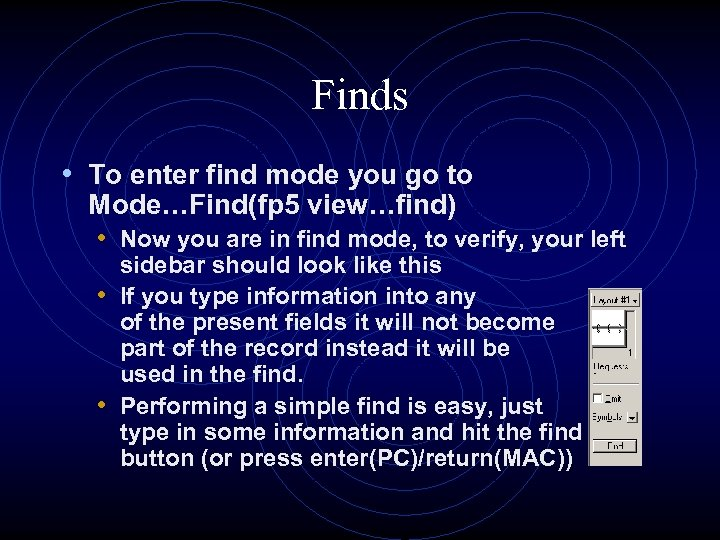Finds • To enter find mode you go to Mode…Find(fp 5 view…find) • Now you are in find mode, to verify, your left sidebar should look like this • If you type information into any of the present fields it will not become part of the record instead it will be used in the find. • Performing a simple find is easy, just type in some information and hit the find button (or press enter(PC)/return(MAC))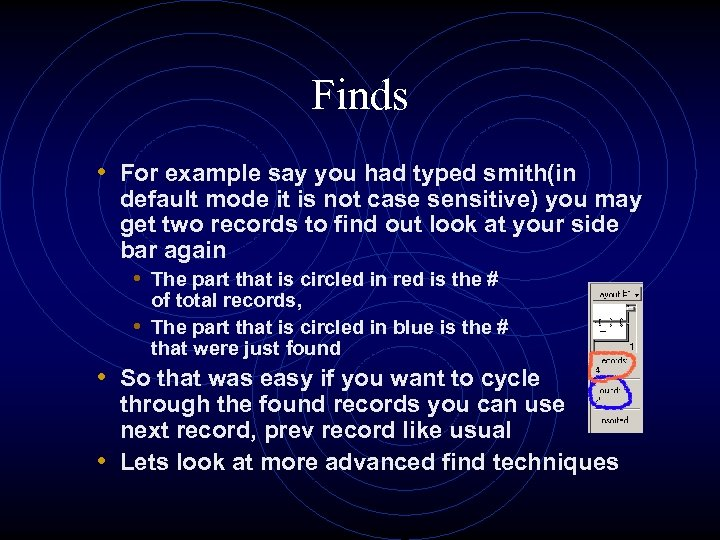Finds • For example say you had typed smith(in default mode it is not case sensitive) you may get two records to find out look at your side bar again • The part that is circled in red is the # of total records, • The part that is circled in blue is the # that were just found • So that was easy if you want to cycle through the found records you can use next record, prev record like usual • Lets look at more advanced find techniques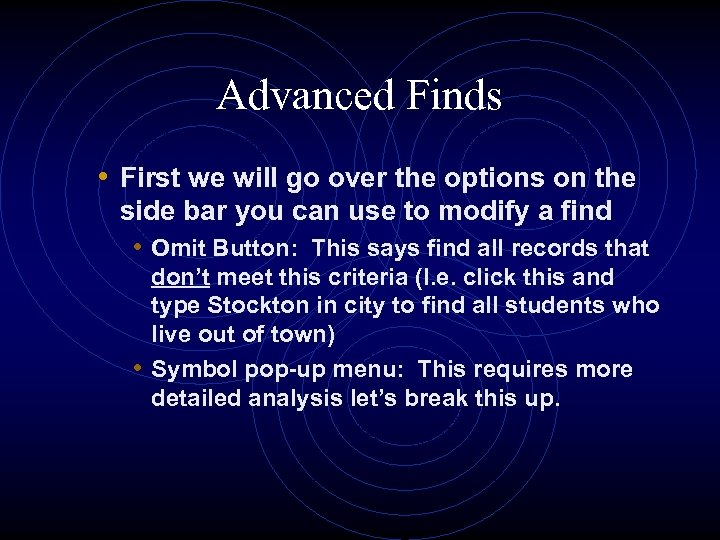Advanced Finds • First we will go over the options on the side bar you can use to modify a find • Omit Button: This says find all records that don’t meet this criteria (I. e. click this and type Stockton in city to find all students who live out of town) • Symbol pop-up menu: This requires more detailed analysis let’s break this up.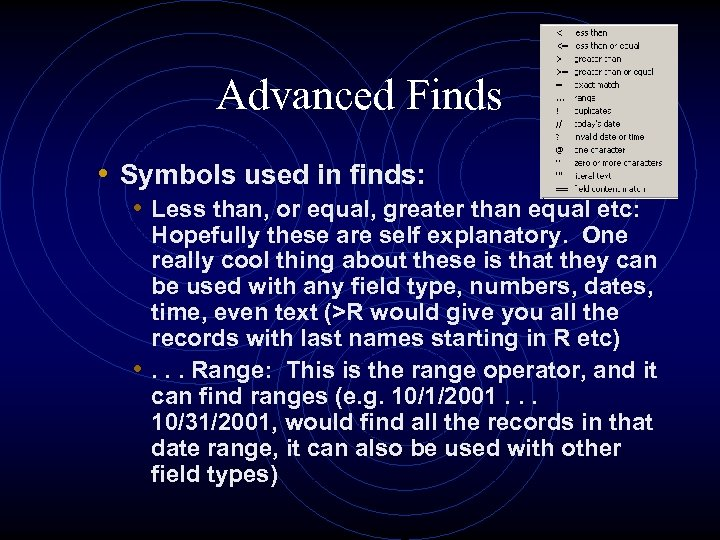Advanced Finds • Symbols used in finds: • Less than, or equal, greater than equal etc: Hopefully these are self explanatory. One really cool thing about these is that they can be used with any field type, numbers, dates, time, even text (>R would give you all the records with last names starting in R etc) • . . . Range: This is the range operator, and it can find ranges (e. g. 10/1/2001. . . 10/31/2001, would find all the records in that date range, it can also be used with other field types)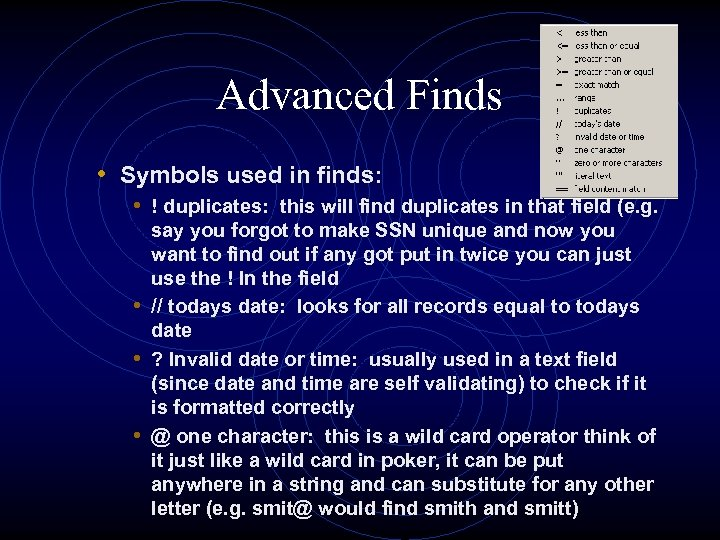Advanced Finds • Symbols used in finds: • ! duplicates: this will find duplicates in that field (e. g. say you forgot to make SSN unique and now you want to find out if any got put in twice you can just use the ! In the field • // todays date: looks for all records equal to todays date • ? Invalid date or time: usually used in a text field (since date and time are self validating) to check if it is formatted correctly • @ one character: this is a wild card operator think of it just like a wild card in poker, it can be put anywhere in a string and can substitute for any other letter (e. g. [email protected] would find smith and smitt)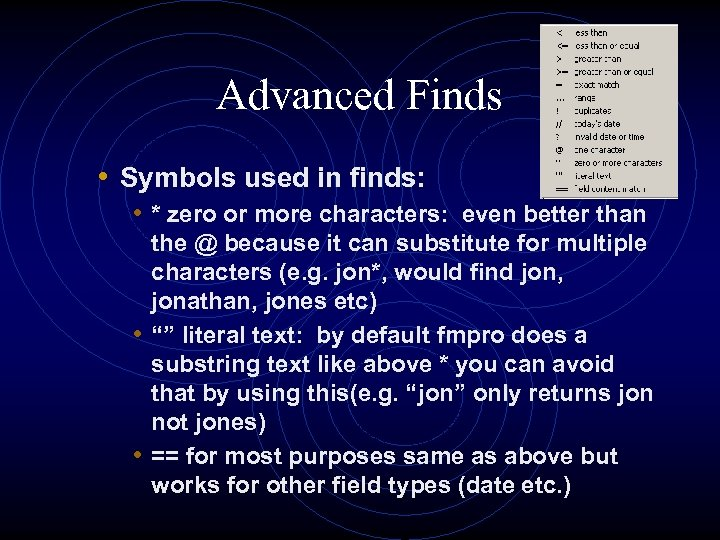Advanced Finds • Symbols used in finds: • * zero or more characters: even better than the @ because it can substitute for multiple characters (e. g. jon*, would find jon, jonathan, jones etc) • “” literal text: by default fmpro does a substring text like above * you can avoid that by using this(e. g. “jon” only returns jon not jones) • == for most purposes same as above but works for other field types (date etc. )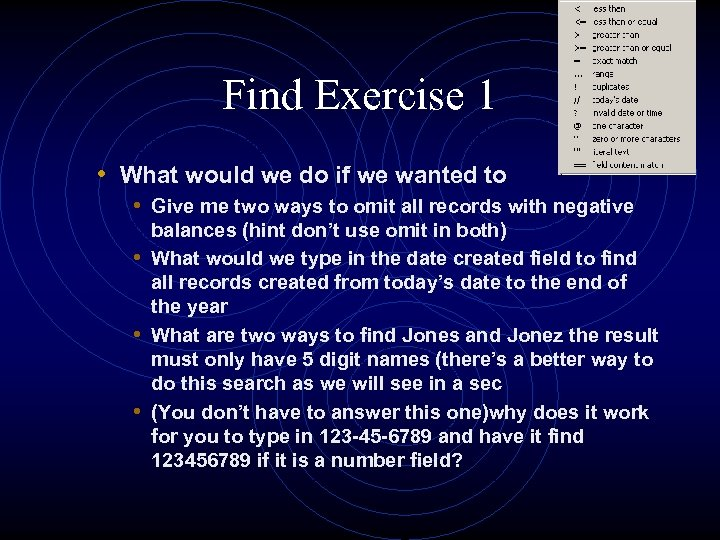Find Exercise 1 • What would we do if we wanted to • Give me two ways to omit all records with negative balances (hint don’t use omit in both) • What would we type in the date created field to find all records created from today’s date to the end of the year • What are two ways to find Jones and Jonez the result must only have 5 digit names (there’s a better way to do this search as we will see in a sec • (You don’t have to answer this one)why does it work for you to type in 123 -45 -6789 and have it find 123456789 if it is a number field?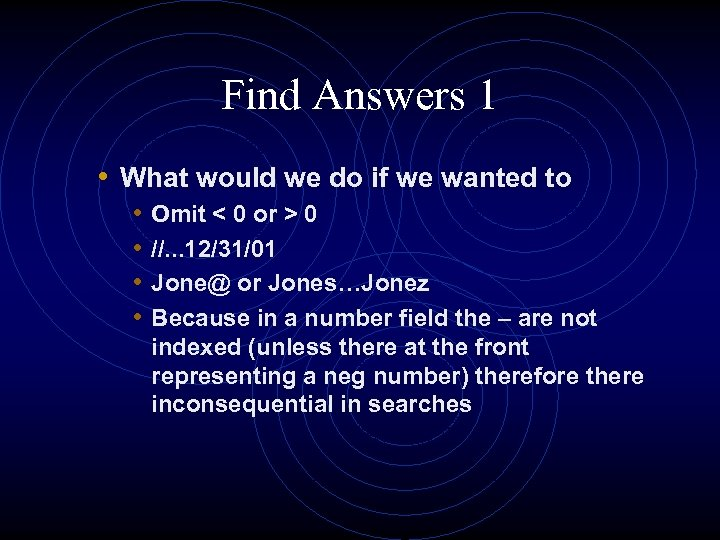Find Answers 1 • What would we do if we wanted to • • Omit < 0 or > 0 //. . . 12/31/01 [email protected] or Jones…Jonez Because in a number field the – are not indexed (unless there at the front representing a neg number) therefore there inconsequential in searches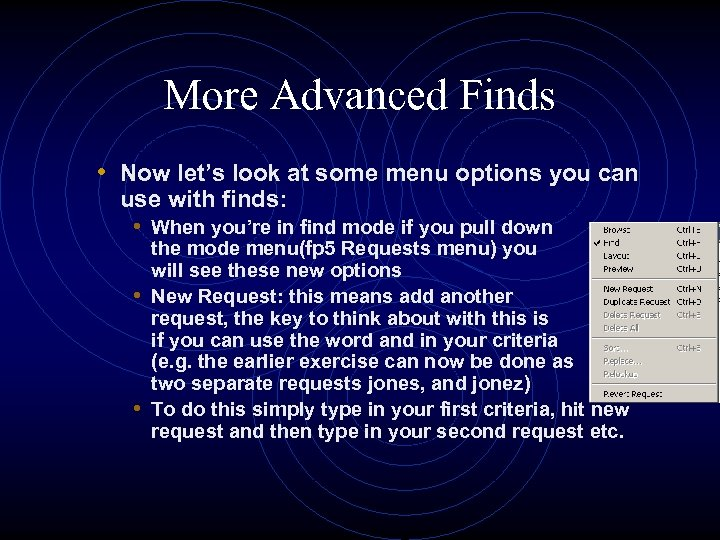More Advanced Finds • Now let’s look at some menu options you can use with finds: • When you’re in find mode if you pull down the mode menu(fp 5 Requests menu) you will see these new options • New Request: this means add another request, the key to think about with this is if you can use the word and in your criteria (e. g. the earlier exercise can now be done as two separate requests jones, and jonez) • To do this simply type in your first criteria, hit new request and then type in your second request etc.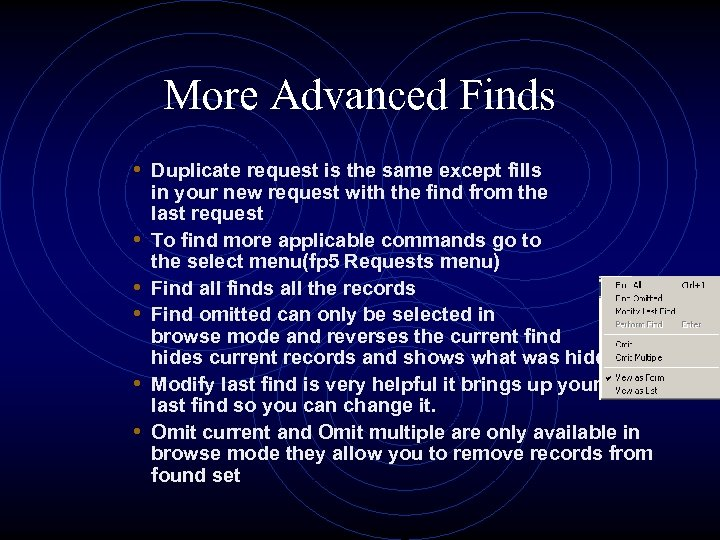More Advanced Finds • Duplicate request is the same except fills • • • in your new request with the find from the last request To find more applicable commands go to the select menu(fp 5 Requests menu) Find all finds all the records Find omitted can only be selected in browse mode and reverses the current find hides current records and shows what was hidden Modify last find is very helpful it brings up your last find so you can change it. Omit current and Omit multiple are only available in browse mode they allow you to remove records from found set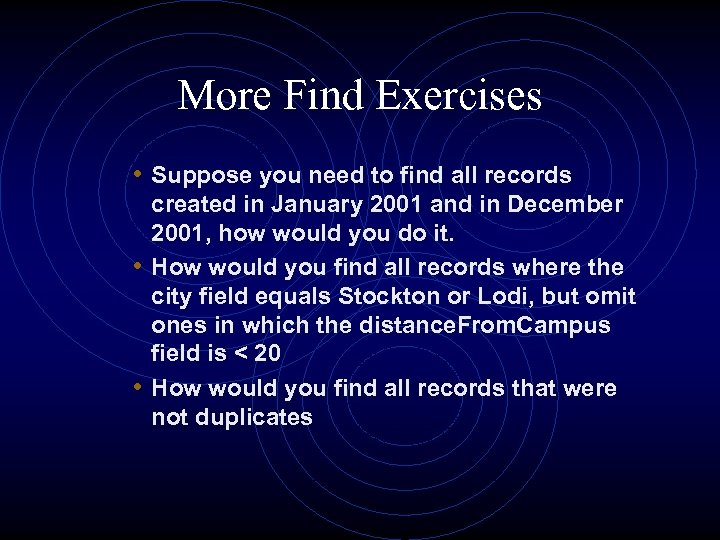More Find Exercises • Suppose you need to find all records created in January 2001 and in December 2001, how would you do it. • How would you find all records where the city field equals Stockton or Lodi, but omit ones in which the distance. From. Campus field is < 20 • How would you find all records that were not duplicates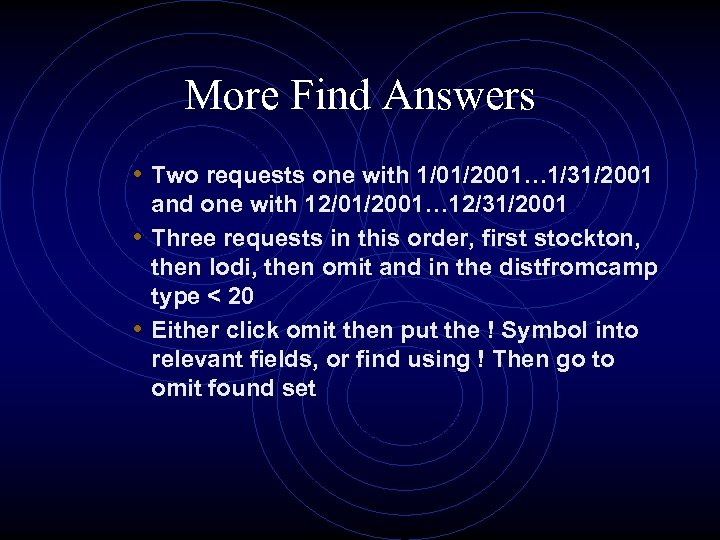More Find Answers • Two requests one with 1/01/2001… 1/31/2001 and one with 12/01/2001… 12/31/2001 • Three requests in this order, first stockton, then lodi, then omit and in the distfromcamp type < 20 • Either click omit then put the ! Symbol into relevant fields, or find using ! Then go to omit found set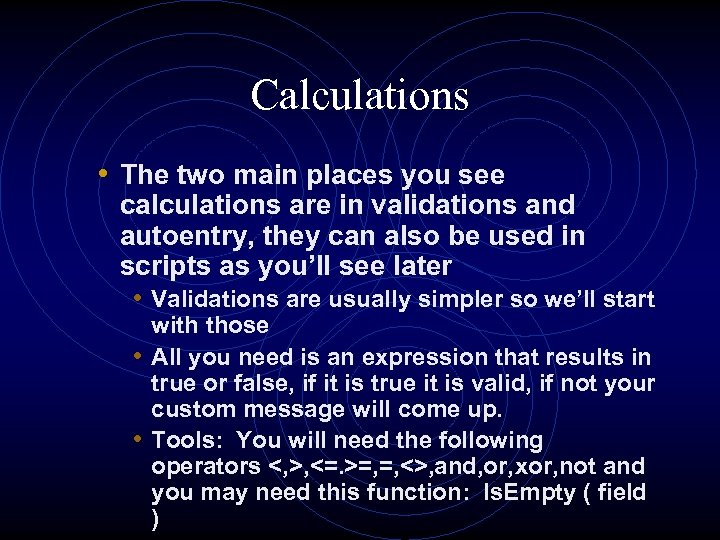Calculations • The two main places you see calculations are in validations and autoentry, they can also be used in scripts as you’ll see later • Validations are usually simpler so we’ll start with those • All you need is an expression that results in true or false, if it is true it is valid, if not your custom message will come up. • Tools: You will need the following operators <, >, <=. >=, =, <>, and, or, xor, not and you may need this function: Is. Empty ( field )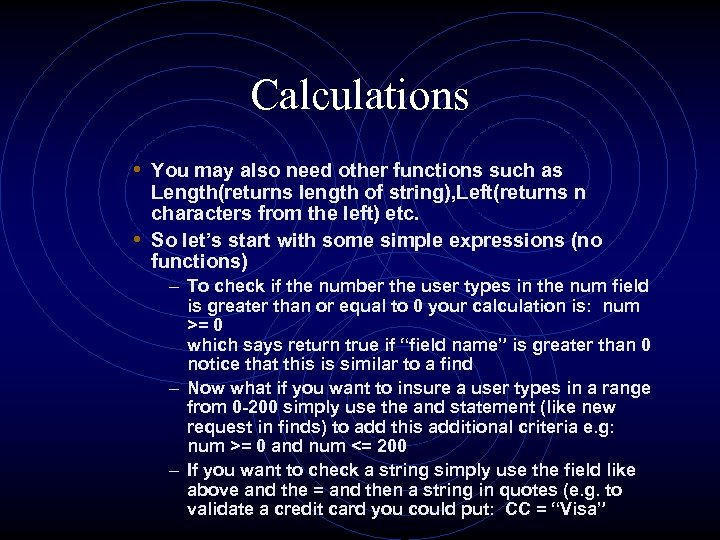Calculations • You may also need other functions such as Length(returns length of string), Left(returns n characters from the left) etc. • So let’s start with some simple expressions (no functions) – To check if the number the user types in the num field is greater than or equal to 0 your calculation is: num >= 0 which says return true if “field name” is greater than 0 notice that this is similar to a find – Now what if you want to insure a user types in a range from 0 -200 simply use the and statement (like new request in finds) to add this additional criteria e. g: num >= 0 and num <= 200 – If you want to check a string simply use the field like above and the = and then a string in quotes (e. g. to validate a credit card you could put: CC = “Visa”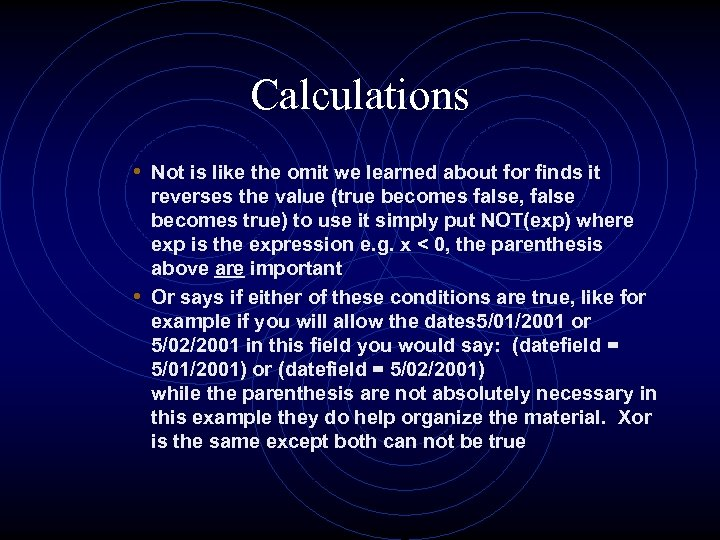Calculations • Not is like the omit we learned about for finds it reverses the value (true becomes false, false becomes true) to use it simply put NOT(exp) where exp is the expression e. g. x < 0, the parenthesis above are important • Or says if either of these conditions are true, like for example if you will allow the dates 5/01/2001 or 5/02/2001 in this field you would say: (datefield = 5/01/2001) or (datefield = 5/02/2001) while the parenthesis are not absolutely necessary in this example they do help organize the material. Xor is the same except both can not be true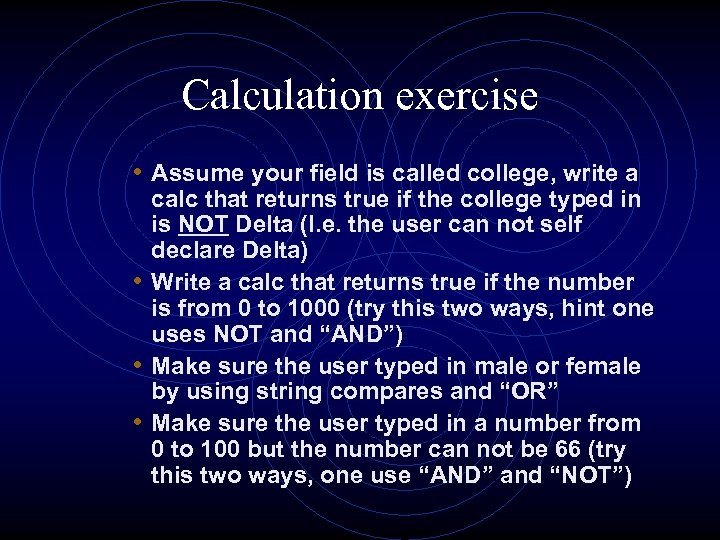Calculation exercise • Assume your field is called college, write a calc that returns true if the college typed in is NOT Delta (I. e. the user can not self declare Delta) • Write a calc that returns true if the number is from 0 to 1000 (try this two ways, hint one uses NOT and “AND”) • Make sure the user typed in male or female by using string compares and “OR” • Make sure the user typed in a number from 0 to 100 but the number can not be 66 (try this two ways, one use “AND” and “NOT”)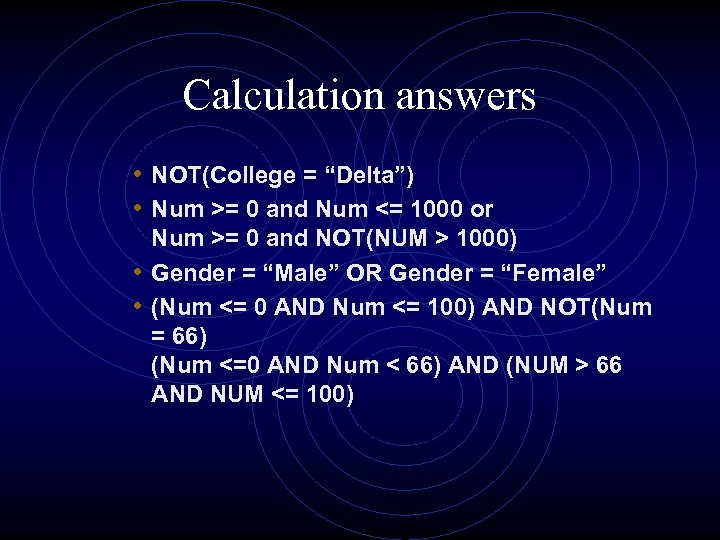Calculation answers • NOT(College = “Delta”) • Num >= 0 and Num <= 1000 or Num >= 0 and NOT(NUM > 1000) • Gender = “Male” OR Gender = “Female” • (Num <= 0 AND Num <= 100) AND NOT(Num = 66) (Num <=0 AND Num < 66) AND (NUM > 66 AND NUM <= 100)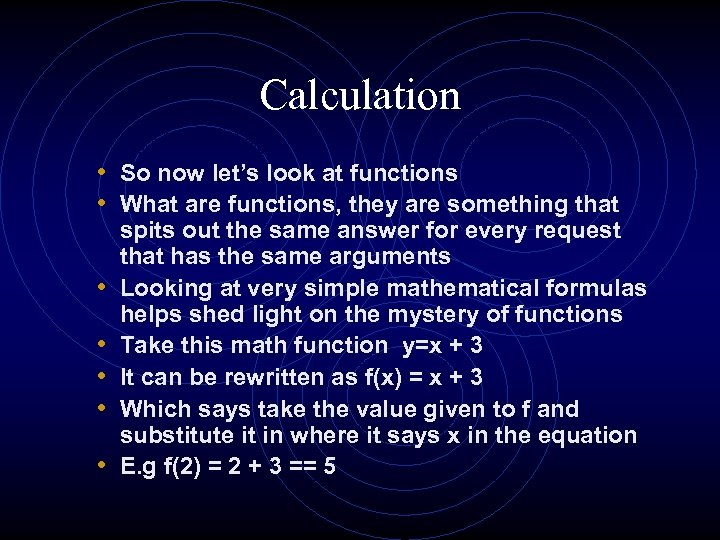Calculation • So now let’s look at functions • What are functions, they are something that • • • spits out the same answer for every request that has the same arguments Looking at very simple mathematical formulas helps shed light on the mystery of functions Take this math function y=x + 3 It can be rewritten as f(x) = x + 3 Which says take the value given to f and substitute it in where it says x in the equation E. g f(2) = 2 + 3 == 5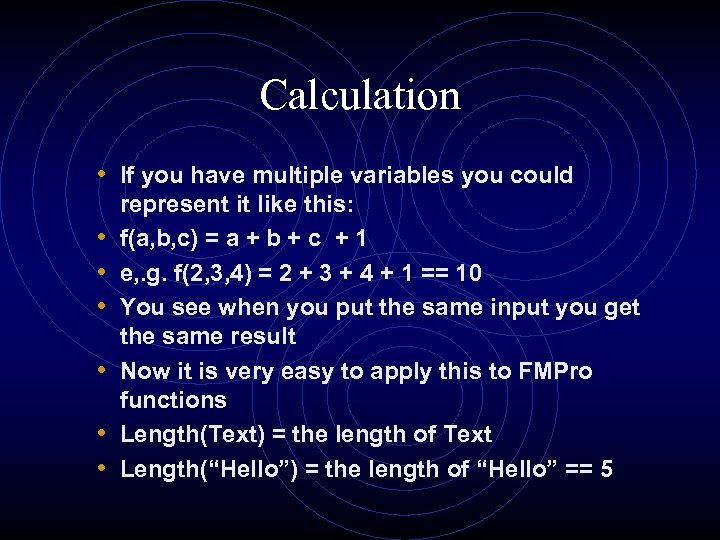Calculation • If you have multiple variables you could • • • represent it like this: f(a, b, c) = a + b + c + 1 e, . g. f(2, 3, 4) = 2 + 3 + 4 + 1 == 10 You see when you put the same input you get the same result Now it is very easy to apply this to FMPro functions Length(Text) = the length of Text Length(“Hello”) = the length of “Hello” == 5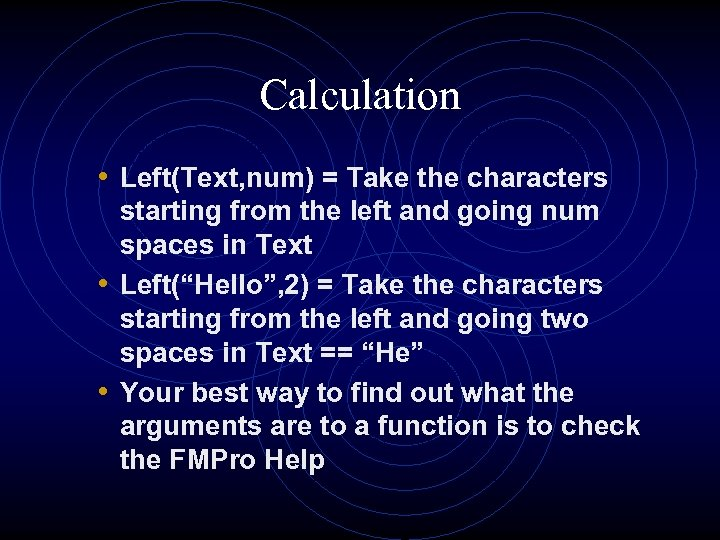Calculation • Left(Text, num) = Take the characters starting from the left and going num spaces in Text • Left(“Hello”, 2) = Take the characters starting from the left and going two spaces in Text == “He” • Your best way to find out what the arguments are to a function is to check the FMPro Help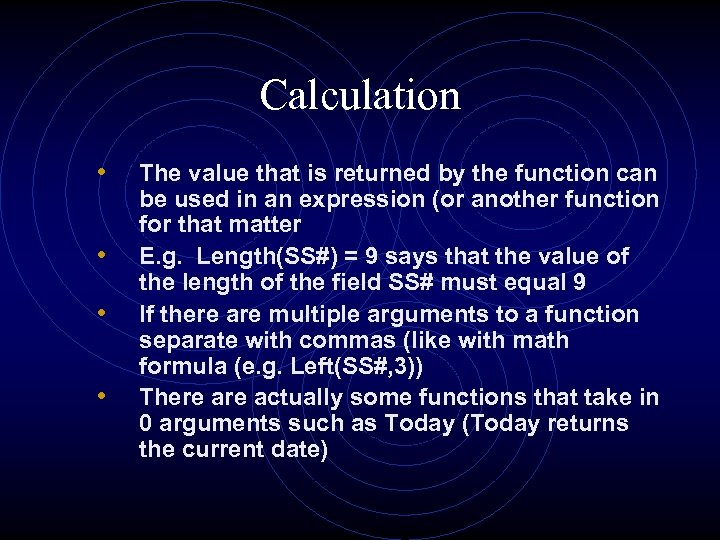Calculation • • The value that is returned by the function can be used in an expression (or another function for that matter E. g. Length(SS#) = 9 says that the value of the length of the field SS# must equal 9 If there are multiple arguments to a function separate with commas (like with math formula (e. g. Left(SS#, 3)) There actually some functions that take in 0 arguments such as Today (Today returns the current date)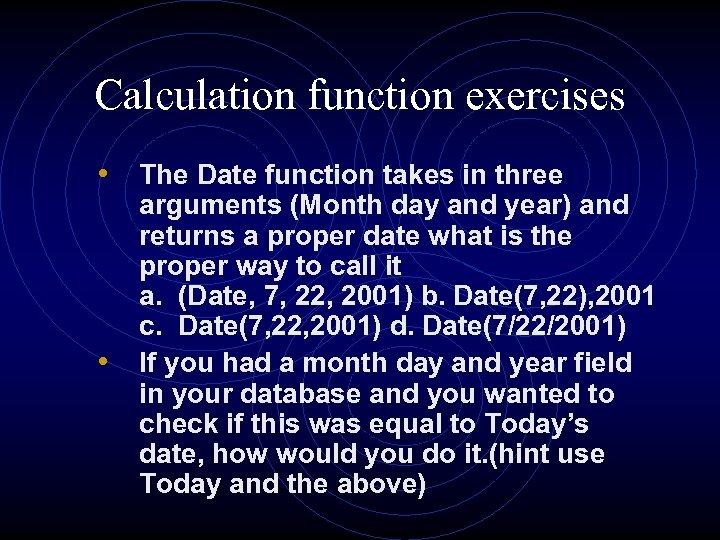Calculation function exercises • The Date function takes in three • arguments (Month day and year) and returns a proper date what is the proper way to call it a. (Date, 7, 22, 2001) b. Date(7, 22), 2001 c. Date(7, 22, 2001) d. Date(7/22/2001) If you had a month day and year field in your database and you wanted to check if this was equal to Today’s date, how would you do it. (hint use Today and the above)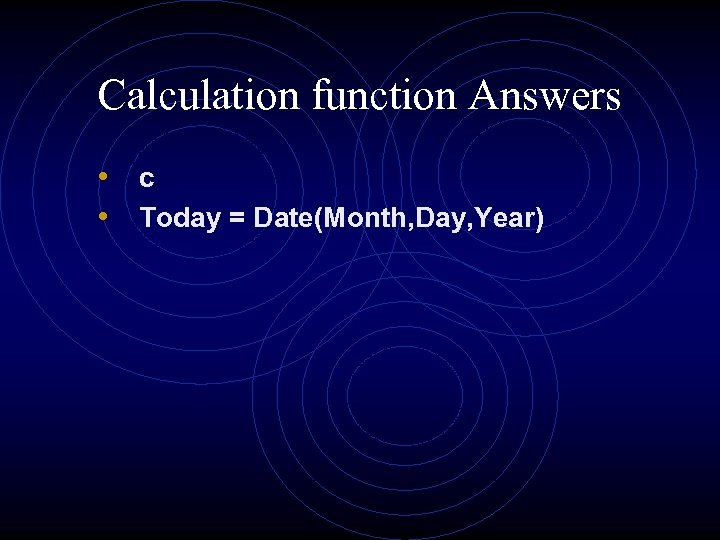Calculation function Answers • c • Today = Date(Month, Day, Year)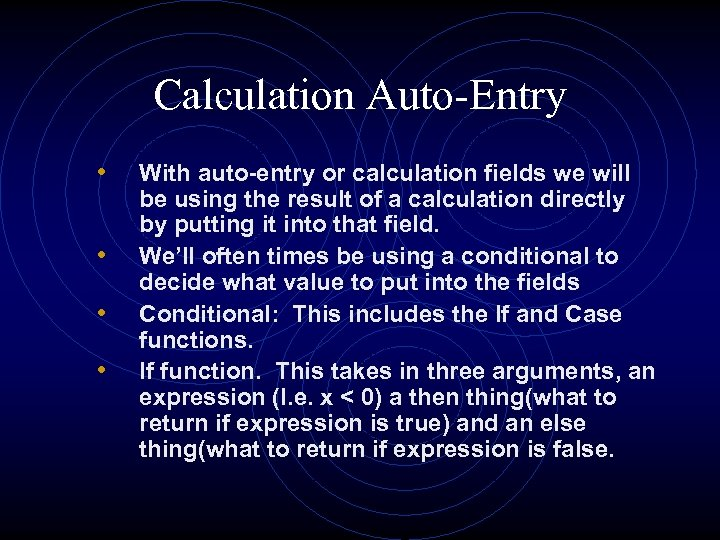Calculation Auto-Entry • • With auto-entry or calculation fields we will be using the result of a calculation directly by putting it into that field. We’ll often times be using a conditional to decide what value to put into the fields Conditional: This includes the If and Case functions. If function. This takes in three arguments, an expression (I. e. x < 0) a then thing(what to return if expression is true) and an else thing(what to return if expression is false.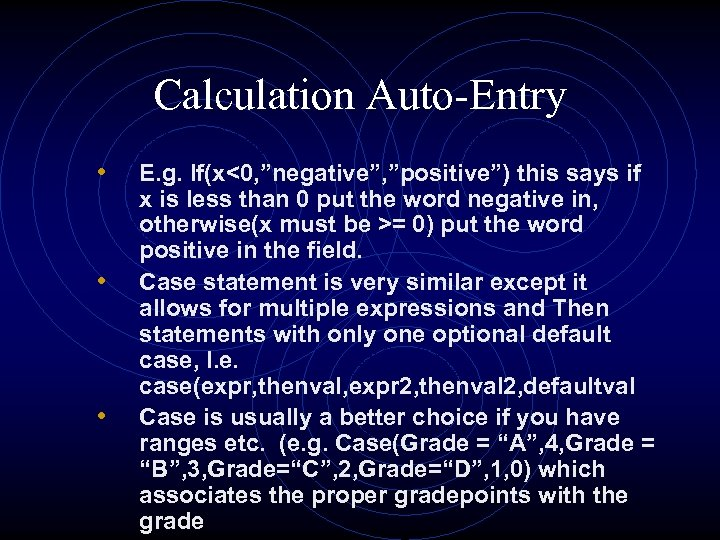Calculation Auto-Entry • • • E. g. If(x<0, ”negative”, ”positive”) this says if x is less than 0 put the word negative in, otherwise(x must be >= 0) put the word positive in the field. Case statement is very similar except it allows for multiple expressions and Then statements with only one optional default case, I. e. case(expr, thenval, expr 2, thenval 2, defaultval Case is usually a better choice if you have ranges etc. (e. g. Case(Grade = “A”, 4, Grade = “B”, 3, Grade=“C”, 2, Grade=“D”, 1, 0) which associates the proper gradepoints with the grade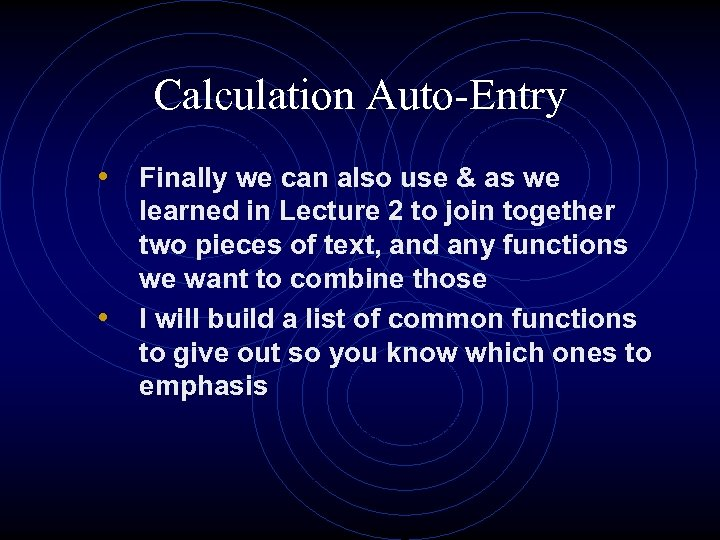Calculation Auto-Entry • Finally we can also use & as we • learned in Lecture 2 to join together two pieces of text, and any functions we want to combine those I will build a list of common functions to give out so you know which ones to emphasis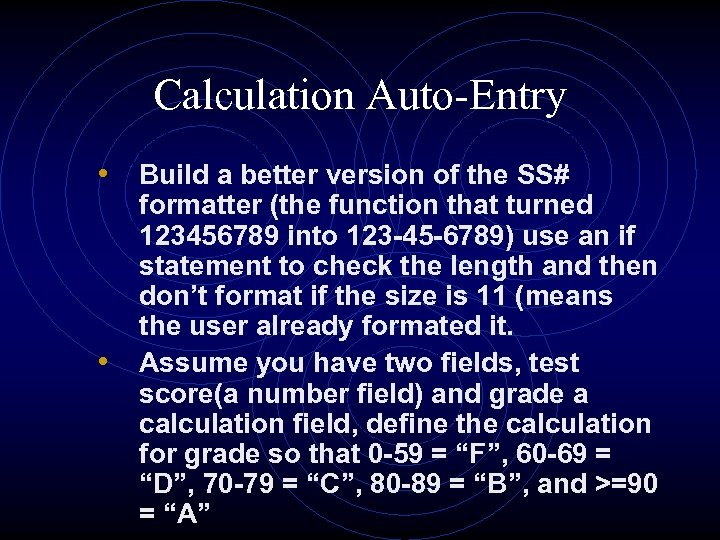Calculation Auto-Entry • Build a better version of the SS# • formatter (the function that turned 123456789 into 123 -45 -6789) use an if statement to check the length and then don’t format if the size is 11 (means the user already formated it. Assume you have two fields, test score(a number field) and grade a calculation field, define the calculation for grade so that 0 -59 = “F”, 60 -69 = “D”, 70 -79 = “C”, 80 -89 = “B”, and >=90 = “A”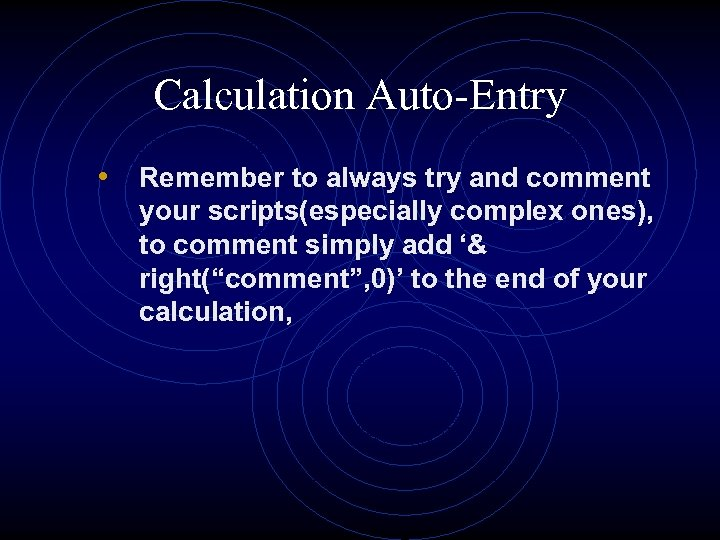Calculation Auto-Entry • Remember to always try and comment your scripts(especially complex ones), to comment simply add ‘& right(“comment”, 0)’ to the end of your calculation,